Next: The branching structure Up: What is branching time Previous: What is branching time

# Introduction

When comparing models or equivalences for concurrent systems, it is common practice to distinguish between linear time and branching time semantics (see for instance DE BAKKER, BERGSTRA, KLOP & MEYER  or PNUELI ). In the former, a process is completely determined by the observable content of its possible (partial) runs, whereas in the latter also the information is preserved where two different courses of action diverge (although branching of identical courses of action may still be neglected). Standard examples are the processes in Figure 1 and 2.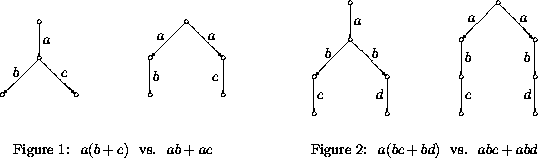In Figure 1, both processes have two complete runs, whose observable content is ab and ac respectively. However, in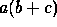these runs diverge after the a-step, whereas in ab+ac they diverge at the onset. This difference can also be described by pointing out thathas a partial run with observable content a that is an initial part of each of the runs ab and ac, whereashas no such run. Hence the two processes are linear time equivalent, but not branching time equivalent. Similarly, the two processes of Figure 2 are identified in linear time semantics but not in branching time semantics, since only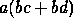has a run a that is an initial part of both of the runs abc and abd.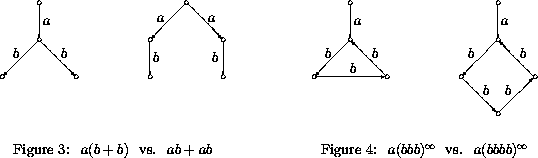Figure 3 illustrates that in branching time semantics only the branching of different courses of action is of importance. The processes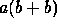and ab+ab are bisimulation equivalent , which is widely considered to be the prime example of a branching time equivalence. Nevertheless, the two runs with observable content ab diverge at a different state. One could employ a stricter notion of branching time, in which also the branching of identical courses of action would be taken into account. Under such a notion, the processes of Figure 3 should be distinguished, but the ones of Figure 4 could safely be identified.

It should be pointed out that branching time is not the definition of a particular model or semantic equivalence, but a criterion that can be satisfied by models or equivalences. A model or equivalence that satisfies this criterion is said to be a branching time model or equivalence, to respect branching time, or to respect the branching structure of processes. Call two processes, represented as labelled transition systems, tree equivalent if their unfoldings into trees are isomorphic. The two processes of Figure 4 for instance are tree equivalent, whereas the ones of Figure 1-3 are not. Tree equivalence is strictly finer than bisimulation equivalence and thus certainly preserves the information on where different courses of action diverge. Hence it constitutes a second example of a branching time equivalence. Moreover, unlike bisimulation equivalence, it would still be a branching time equivalence under the stricter interpretation of branching time contemplated above.

For processes without silent moves, it is commonly agreed that an equivalence respects branching time iff it is finer than or equal to bisimulation equivalence. Hence this yield a candidate for a formal definition of the concept of a branching time equivalence. However, in the presence of silent moves, or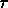-steps, the situation is less clear. In VAN GLABBEEK & WEIJLAND  it is argued that branching bisimulation, that in the absence of silent moves coincides with plain bisimulation, takes over this rôle of bisimulation in the presence of's. However, this is not inherent in the definition of the equivalence, and was meant to be a result rather than a definition. The reasoning of  is illustrated in Figures 5 and 6.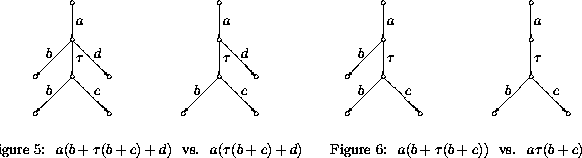The first process in Figure 5 has a run with observable content ab, that diverges from the run with observable content ac at the same point where it diverges from the run ad. Thus it does not pass through a state in which it is still possible to continue with a course of action that involves a c, but not possible to continue with a course of action involving a d. Such a run is not present in the other process. It follows that these processes have a different branching structure and can not be identified by a branching time equivalence. As MILNER's notion of weak bisimulation  identifies both processes, it does not respect branching time. The notion of branching bisimulation on the other hand gives rise to a finer equivalence that does distinguish the processes of Figure 5. The processes of Figure 6 are branching bisimulation equivalent however, and indeed no argument can be construed that would indicate disrespect of branching time, the key argument being that the various runs with observable content ab can be regarded as the same course of action.

The best definition of a branching time equivalence so far is perhaps the one implicit in the notion of a consistent colouring, introduced in : An equivalence respects branching time if the colouring on processes/states, obtained by giving equivalent processes the same colour, is consistent in the sense of . Here a sequence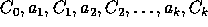is a concrete coloured trace of a process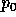if there is a run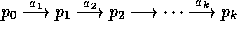, such that processhas colour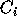; a coloured trace of p is a sequenceobtained from a concrete coloured trace of p by replacing any subsequence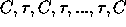by C; and a colouring is consistent if processes with the same colour have the same set of coloured traces. The idea behind a consistent colouring is that processes with the same colour have the same potential of further courses of action. A coloured trace indicates how these potentials vary (diminish) during a run. As expected, under this definition branching bisimulation turns out to be the coarsest branching time equivalence.

Although this definition captures the concept of branching time reasonably well, it has the disadvantage of being tailored to a setting with silent moves (through the reduction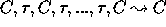). What would be more convincing would be a definition that in no way refers to internal actions, and still yields branching bisimulation as the coarsest equivalence respecting the branching structure of processes. Moreover, it would be nice to know what precisely the branching structure of a process is. This is the goal of the present paper. I will formally define the branching structure of a process, and declare an equivalence to respect branching time iff it only relates processes with the same branching structure. This will be the case iff the equivalence is finer than or equal to branching bisimulation equivalence. Similarly a model of concurrency respects branching time iff processes that are represented by the same semantic object have the same branching structure.Next: The branching structure Up: What is branching time Previous: What is branching time

Rob van Glabbeek